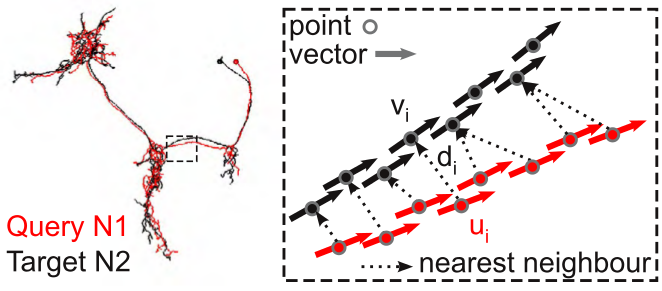NBLAST works by decomposing neurons into point and tangent vector representations. One neuron is designated the query and the other the target. For each query segment (defined by a midpoint and tangent vector) the nearest neighbor (using straight-forward Euclidean distance) is identified in the target neuron. A score for the segment pair is calculated as a function of two measurements: d, the distance between the matched segments (indexed by i), and u_i . v_i, the absolute dot product of the two tangent vectors; the absolute dot product is used because the orientation of the tangent vectors has no meaning in our data representation (see image).The scores are then summed over each segment pair to give a raw score, S:

S = sum of f(d, u_i . v_i)

To determine a suitable f, we developed an approach inspired by the scoring system of the BLAST algorithm. For each segment pair we defined the score as the log probability ratio:

f = log_2 p_match / p_rand

i.e. the probability that the segment pair was derived from a pair of neurons of the same type, versus a pair of unrelated neurons.

We then defined p_match empirically by considering 150 olfactory projection neurons innervating the same glomerulus. p_rand was calculated simply by drawing 5,000 random pairs of neurons from the database, assuming that the large majority of such pairs are unrelated neurons. Joint distributions were calculated using 10 bins for the absolute dot product and 21 bins for the distance to give two 21 row by 10 column matrices. The 2D histograms were then normalized to convert them to probabilities and the log ratio defined the final scoring matrix.